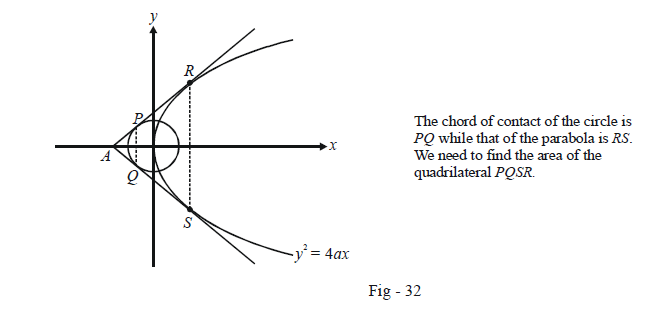# Examples on Tangents and Chords of Parabolas Set 1

Go back to  'Parabola'

Example – 31

Find the locus of the mid-points of the normal chords of the parabola $${y^2} = 4ax$$ .

Solution: Let P(h, k) be the point whose locus we wish to determine. If a chord passing through P is bisected at P, then its equation is, as discussed earlier,

\begin{align}& \qquad \;\;T(h,k) = S(h,k)\\\\&\Rightarrow \quad yk - 2a(x + h) = {k^2} - 4ah\\\\&\Rightarrow \quad ky - 2ax = {k^2} - 2ah \qquad \dots \left( 1 \right)\end{align}

Since this chord is also a normal to the same parabola, its equation can also be written as

$y - mx = - 2am - a{m^3} \qquad \dots {\rm{ }}\left( 2 \right)$

(1) and (2) represent the same chord in two different forms, so that

$\frac{k}{1} = \frac{{2a}}{m} = \frac{{{k^2} - 2ah}}{{ - 2am - a{m^3}}}$

m can easily be eliminated using these relations to obtain a relation in h and k as

${k^2}({k^2} - 2ah) + 4{a^2}({k^2} + 2{a^2}) = 0$

Thus, the required locus is

${y^2}({y^2} - 2ax) + 4{a^2}({y^2} + 2{a^2}) = 0$

Example - 32

From a point A common tangents are drawn to the circle {x^2} + {y^2} = \begin{align}\frac{{{a^2}}}{2}\end{align} and the parabola $${y^2} = 4ax.$$ Find the area of the quadrilateral formed by the common tangents, the chord of contact of the circle and the chord of contact of the parabola.

Solution: The figure below makes the situation described in the question clearer:Any tangent to $${y^2} = 4ax$$ can be written in terms of its slope m as

\begin{align}&\qquad \quad \;\;y = mx + \frac{a}{m}\\\\&or \qquad \;\;{m^2}x - my + a = 0\end{align}

If this is to touch the circle  {x^2} + {y^2} = \begin{align}\frac{{{a^2}}}{2}\end{align}, its distance from the circle’s centre (0, 0) must be equal to the circle’s radius \begin{align}\frac{a}{{\sqrt 2 }}.\end{align}

Thus,

\begin{align}& \qquad \; \frac{a}{{\sqrt {{m^4} + {m^2}} }} = \frac{a}{{\sqrt 2 }}\\\\&\Rightarrow \quad {m^4} + {m^2} = 2\\\\&\Rightarrow \quad ({m^2} + 2)({m^2} - 1) = 0\\\\&\Rightarrow \quad m = \pm 1\end{align}

Thus, the two tangents from A are $$y = \pm (x + a)$$ so that the point A is (–a, 0).

The chord of contact PQ is

\begin{align}& \qquad \;\; {T_C}( - a,0) = 0\\&\Rightarrow \quad - ax + 0y = \frac{{{a^2}}}{y}\\&\Rightarrow \quad x = - \frac{a}{2}\end{align}

The chord of contact RS is

\begin{align}& \qquad \;\; {T_P}( - a,0) = 0\\\\&\Rightarrow \quad 0y = 2a(x - a)\\\\&\Rightarrow \quad x = a\end{align}

The length PQ is now simply a (verify) whereas RS becomes the Latus-rectum (since x = a for RS)

so that its length is 4a. The distance between PQ and RS is \begin{align}\frac{a}{2} + a = \frac{{3a}}{2}.\end{align}

Thus, the area of PQSR (note that it is a trapezium) is

\begin{align}\Delta&= \frac{1}{2}(a + 4a) \times \frac{{3a}}{2}\\\\&= \frac{{15{a^2}}}{4}{\rm{ sq}}{\rm{. units}}\end{align}

Example - 33

Find the locus of an external point P from which exactly two distinct normals can be drawn to the parabola $${y^2} = 4ax.$$

Solution: The equation of the normal in terms of slope is a cubic, from which we inferred that in general three normals can be drawn to the parabola from a given point. Thus, we could either have one real and two imaginary solutions of the cubic (implying only one normal) or all the three roots of the cubic could be real (implying three real normals).

In this question, we have exactly two distinct normals which can only happen if all the three roots of the cubic are real and two of these three roots are identical. This is the insight we use for solving this question.

The equation of an arbitrary normal to the parabola is

$y = mx - 2am - a{m^3}$

If this passes through $$P(h,k),$$ we have

\begin{align}&\qquad\quad k = mh - 2am - a{m^3}\\\\&\Rightarrow \quad {m^3} + (2a - h)m + k = 0 \qquad \qquad\qquad \dots \left( 1 \right)\end{align}

Let the roots of this cubic be $$m{_1},{m_1}{\rm{ }}\;and \;{ m_2}$$ so that

\begin{align}&2{m_1} + {m_2} = 0 \quad \Rightarrow \quad {m_2}\;=\; - 2{m_1}\\\\&m_1^2{m_2} = - \frac{k}{a}\end{align}

These two relations give

m_1^3 = \frac{k}{{2a}} \quad \Rightarrow \quad{m_1} \;=\; {\left( {\begin{align}\frac{k}{{2a}}\end{align}} \right)^{\frac{1}{3}}}

We now substitute this value of $${m_1}$$ back in (1) to obtain a relation between h and k :

\begin{align}& \;\qquad \frac{{ak}}{{2a}} + (2a - h){\left( {\frac{k}{{2a}}} \right)^{\frac{1}{3}}} + k = 0\\\\&\Rightarrow \quad 27a{k^2} = 4{(h - 2a)^3}\end{align}

Using (x, y) instead of (h, k) gives the required locus as

$27a{y^2} = 4{(x - 2a)^3}$